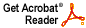# Hausdorff measure and Carleson thin sets

Joel H. Shapiro

Proc. Amer. Math. Soc. 79 (1980), 67--72

Abstract: Let H be the Hausdorff measure on the unit circle induced by the determining function h defined on (0,1], where h(t)/t is strictly decreasing. It is shown here that a necessary and sufficient condition for every Carleson subset of the circle to be H-null is that 1/h not be integrable on (0,1]. Thus non-integrability of 1/h is necessary and sufficient for every positive Borel measure m on T with modulus of continuity O(h(t)) to place zero mass on every Carleson set.

After this paper was accepted for publication I learned that its results had already been obtained by Patrick Ahern in The mean modulus and derivative of an inner function , Indiana Univ. Math. J. 28 (1979), 311--347, in particular Lemma 2.3 and Theorem 2.3 of that paper.

Download .pdf file (900KB)